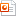검색어 입력폼

# Representation and Approximation in Vector Space

저작시기 2008.09 | 등록일 2008.10.17MS 파워포인트 (ppt) | 27페이지 | 가격 1,500원

## 소개글

Mathematical Methods and Alogorithms for Signal Prpcessing 책에 chapter 3장인
Representation and Approximation in Vector Space의 내용중 일부를 정리한 내용인데요

Hilbert space상에서 vector 내용들을 다루었습니다.

간단한 벡터와 벡터 사이에서의 orthogonal principle로 증명하고 error minimum을 정의하고
여러가지 알고리즘을 이용하여 Approximation을 유도하고
Least square(LS)와 Minimum mean square(MMS)유도합니다.
둘의 차이점을 비교하는 것으로 끝을 냅니다.

# 책에 관계되서 발표하시는 분이 쓰시면 좋을꺼 같구요

# 아니면 관계된 내용 발표하시는 분이면서 Linear algebra, vector에 관계이론을 공부하시는
분이 쓰시면 좋을꺼 같습니다.

## 목차

3.1 The approximation problem in Hilbert space
3.1 Find coefficients to minimize error (one vector)
3.1 Find coefficients to minimize error (two vector)
3.1 Find coefficients to minimize error (vectors)
3.2 The orthogonality principle
Appendix E 1.1 Derivatives and Gradients
3.4 Matrix representations of least-square problems
3.5 Minimum error in vector-space approximations
3.6 Approximation by continuous polynomials
3.7 Approximation by discrete polynomials
3.8 Linear regression
3.9 Least-squares filtering
3.10 Minimum mean-square estimation
3.11 Minimum mean-square error (MMSE) filtering
3.12 Comparison of least-squares and minimum mean-squares

## 본문내용

3.1 The approximation problem in Hilbert space

Let..
.. S be a normed linear vector space
.. be a set of linearly independent vector in a vector space S
.. V = span(T)
.. , find the coefficients c1,c2,…,cm

 , is approximation
 , e is approximation error

3.2 The orthogonality principle

Theorem
Let p1, p2, …,pm be data vector in a vector space S
Let x be any vector in S
In the representation…

The induced norm of the error vector is minimized when the error is orthogonal to each of the data vectors,

## 참고 자료

Mathematical Methods and Alogorithms for Signal Prpcessing - ToddK. Moon Wynn C, Stirling